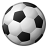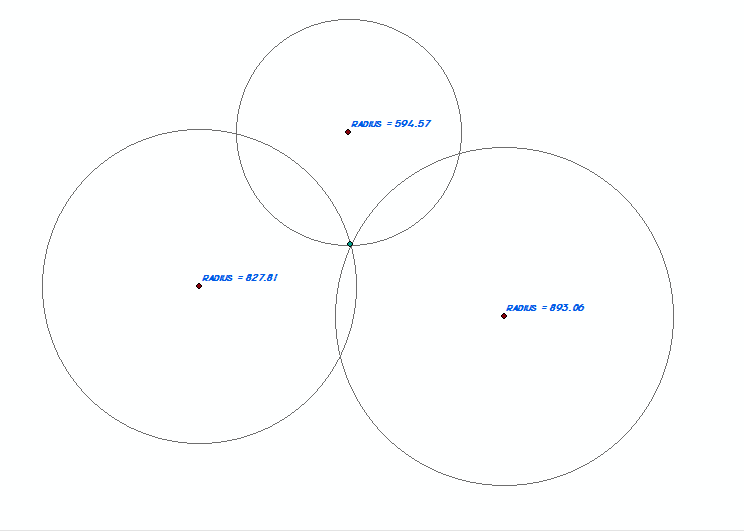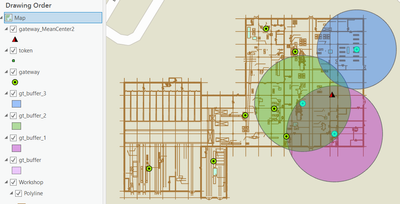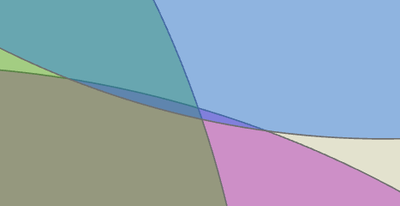# Trilateration in ArcMap

1805
4
12-17-2016 02:21 PMNew Contributor

Hi I am trying to find a way to natively trilateral between three points in ArcMap, using the Distance-Distance construction feature I can do this with two measurements but is there a way to give a third input measurement?

I've also tried buffering each point by the distance and then running an intersect but the issue is, especially with hand collected data, if the records are not 100% accurate you are left with a "cocked hat" where the three circles do not intersect so the point has to be placed manually. this is also quite time intensive when you have multiple fixes to plot.

4 RepliesRegular Contributor II

Use Mean center tool. mean center

Then use Point Distance tool to determine the distances from mean center to all 3 points .

point distanceNew Contributor

Hi Abullah

This would assume that the the distances are all equally distant from each other which they are not. This is the maths behind trilateration: Trilateration - WikipediaRegular Contributor II

This would assume that the the distances are all equally distant from each other which they are not.

????

Mean center is not the equal distance from each other points.

the following picture is the Mean Center to random 3 points.I think that you want to do 3 circles intersect each other and not Trilateration.

Trilateration is not as your post picture.look for intersection in 3 circles,it is an area and not a point as you want.

where the three circles do not intersect so the point has to be placed manually

.New Contributor

Mean Center tool doesn't calculate the Trilateration point, see below; is the center point of the triangle (base on weights) whereas the intersection point(or area) is a bit to the sideany other GP tools to recommend?

P.S. cannot use buffers intersect as sometimes there's 0 solutions.